# Hiking accessories

49 products found
Filtres

Gender

Size

Price

€10.00 - €70.00

Couleurs

Sport

Use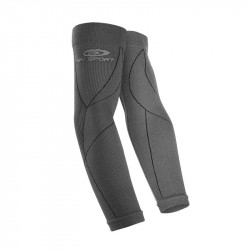• S/M
• L/XL• S/M
• L/XL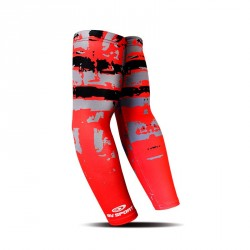• L/XL• L/XL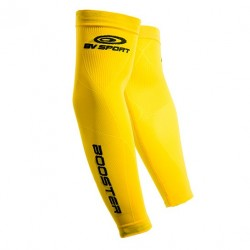• L/XL• L/XL• L/XL• S/M
• L/XL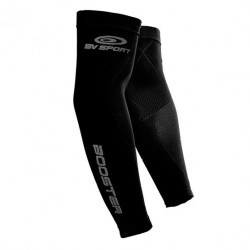• S/M
• L/XL• L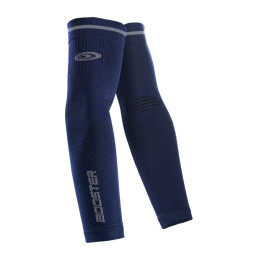• S
• M
• L• S
• M
• L• S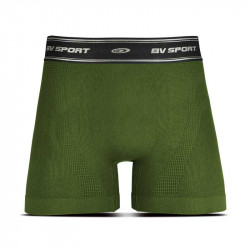• S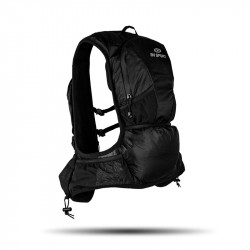• One Size• One Size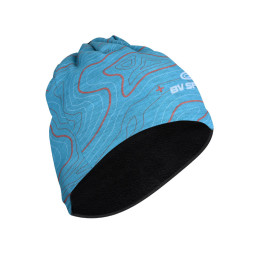• One Size-€12.05
• One Size• One Size• One Size• One Size• One Size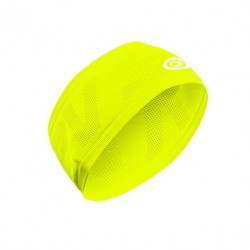• One Size• One Size• One Size• One Size• One Size• One Size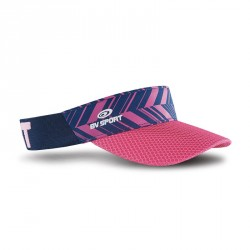• One Size• One Size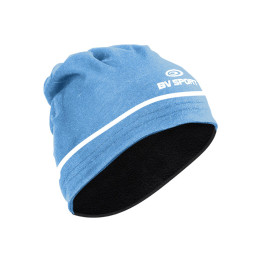• One Size• One Size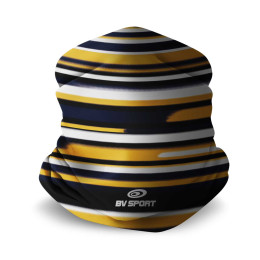• One Size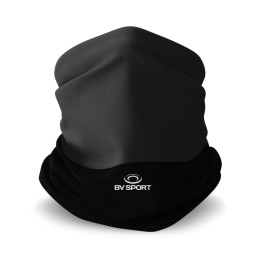• One Size• One Size• One Size